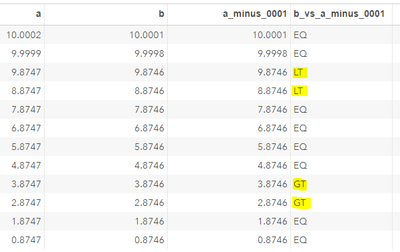## Unexpected flagging when doing simple calculation

Hi Guys,

I’ve created some stand-alone code in a test file to illustrate some unexpected behavior that I am getting. We have 2 values, ‘a’ and ‘b’, with the ‘b’ column equal to the value of a-0.0001

I then create a flag to compare the column b with a calculation of a-0.0001 and flag as LT, EQ or GT b. Here is the stand-alone test data and code:

data test;
input a b;
datalines;
10.0002 10.0001
9.9999 9.9998
9.8747 9.8746
8.8747 8.8746
7.8747 7.8746
6.8747 6.8746
5.8747 5.8746
4.8747 4.8746
3.8747 3.8746
2.8747 2.8746
1.8747 1.8746
0.8747 0.8746
;
run;

data test;
set test;
a_minus_0001=a-0.0001;
if b lt a-0.0001 then b_vs_a_minus_0001='LT';
else if b eq a-0.0001 then b_vs_a_minus_0001='EQ';
else if b gt a-0.0001 then b_vs_a_minus_0001='GT';
run;

The result is quite strange/terrifying. As you can see, all results should flag as EQ, but some flag as GT and others flag as LT:If anyone could have a little look at this and see if they can add any info. We get the correct results if we force a round to 4 decimal places, but that would not be something that you would intuitively so. I'm stumped with this functionality…

Any help gratefully appreciated.

Thank you.

2 REPLIES 2

## Re: Unexpected flagging when doing simple calculation

Digital computers cannnot represent most decimal numbers exactly. So that's why you get the result you see. If, however, you use the FUZZ function, the results should make sense. The ROUND function can work here as well.

``````data test1;
set test;
if fuzz(b-(a-0.0001)) < 0 then b_vs_a_minus_0001='LT';
else if fuzz(b-(a-0.0001)) = 0 then b_vs_a_minus_0001='EQ';
else if fuzz(b-(a-0.0001)) > 0 then b_vs_a_minus_0001='GT';
run;``````

See here for a more detailed discussion of the matter:

Machine Precision

Machine Precision part 2

--
Paige Miller

## Re: Unexpected flagging when doing simple calculation

Hi @ShaneConnolly and welcome to the SAS Support Communities!

You have already got a correct solution. Let me just add a few bits (i.e., binary digits) ...

I like your example values with the .8747 decimals because they demonstrate that not only the decimals are relevant for the computer's result of the subtraction, but also the order of magnitude of the numbers in the binary system. For instance, 2.8747 and 3.8747 fall into the (right-open) interval [2**1, 2**2) and hence are of the same order of magnitude in the binary system.  However, 4.8747, 5.8747, 6.8747 and 7.8747 belong to the next higher order of magnitude: [2**2, 2**3). Finally, 8.8747 and 9.8747 share yet another order of magnitude: [2**3, 2**4). This is the reason why the flag values (in variable b_vs_a_minus_0001) are the same within each of these three groups, but possibly differ between the groups.

All of the numbers in your code are among those mentioned by Paige Miller that are not exactly representable in the computer's memory (here: in the 64 bits of a numeric SAS variable of length 8 bytes) because they are periodic fractions in the binary system. This is true for 99.84% (!) of the numbers with up to four decimal places, hence no surprise here. (The repeating digit patterns of those periodic fractions have a length of 500 bits in each of your examples, so you cannot recognize the repetition when looking at only 64 bits.)

The problem starts when the binary numbers are rounded to 53 bits (52 mantissa bits plus the so called "implied bit") to fit into the 8 bytes (=64 bits), 12 bits of which are reserved for the sign and the exponent (corresponding to the order of magnitude; assuming a Windows or Unix operating system), see Numerical Accuracy in SAS Software:

```exact   0.8747:     1.1011111111011000101011011010101110011111010101011001101... * 2**-1
rounded 0.8747:     1.1011111111011000101011011010101110011111010101011010       * 2**-1 (rounded up)
rounded 1.8747:    1.1101111111101100010101101101010111001111101010101101        * 2**0  (rounded up)
rounded 2.8747:   1.0110111111110110001010110110101011100111110101010110         * 2**1  (rounded down)
rounded 3.8747:   1.1110111111110110001010110110101011100111110101010110         * 2**1  (rounded down)
rounded 4.8747:  1.0011011111111011000101011011010101110011111010101011          * 2**2  (rounded down)
rounded 5.8747:  1.0111011111111011000101011011010101110011111010101011          * 2**2  (rounded down)
rounded 6.8747:  1.1011011111111011000101011011010101110011111010101011          * 2**2  (rounded down)
rounded 7.8747:  1.1111011111111011000101011011010101110011111010101011          * 2**2  (rounded down)
rounded 8.8747: 1.0001101111111101100010101101101010111001111101010110           * 2**3  (rounded up)
rounded 9.8747: 1.0011101111111101100010101101101010111001111101010110           * 2**3  (rounded up)```

Highlighted in blue are the bits occupied by the integer part of the decimal numbers. As you can see, the place where the rounding occurs depends on the order of magnitude of the number (rounded-up digits are highlighted in red). The rounding error propagates when 0.0001 is subtracted. Finally, the result of the subtraction (done at a greater precision) is rounded and, again, it depends on the order of magnitude of the result, where exactly the rounding occurs.

Here is what the subtraction 9.8747−0.0001 looks like internally:

``` 1.0011101111111101100010101101101010111001111101010110                  * 2**3
-0.000000000000000011010001101101110001011101011000111000100001100101101 * 2**3
=1.001110111111110010111001001000111010001010011100011111011110011010011 * 2**3
Rounded up to:
1.0011101111111100101110010010001110100010100111001000                  * 2**3```

However, the correct representation of 9.8746 is

``` 1.0011101111111100101110010010001110100010100111000111 * 2**3, rounded down from
1.001110111111110010111001001000111010001010011100011101111001101001101... * 2**3```

which explains the "LT" flag you obtained for this case.

The other results can be explained analogously.

Discussion stats
• 2 replies
• 228 views
• 1 like
• 3 in conversation